### 1. Create exam

(1) Tap the [+] button to create a exam.

(2) Enter [Exam name].

(3) Select [Category].

You can select [Workbook], [Trial exam] or [Final exam].

Exams contains several answer sheet samples. If you can not find the answer sheet for the desired exam, please create a new answer sheet by tapping [Create another answer sheet]. For the creation of the answer sheet, see “3. Preparation of the answer sheet”. For the creation of the answer sheet, see “3. Create answer sheet“.

(5) Tap [SAVE] to save the exam and show the [Start exam] screen.(b) Close [Edit Exam] screen.
(c) Create a new answer sheet. It is used when you can not find the answer sheet of the desired exam on the answer sheet of (4).

### 2. Start exam

(1) Tap [START] button.

The tapped symbol becomes gray. If you do not know the question, you can tap [?]. Then the question is answered. You do not have to use [?] in particular.

(3) When you finish all the answers to the exam, tap [FINISH] button.

A confirmation dialog is shown.

(4) Tap [SCORE EXAM] button to score the exam.

The tapped symbol is surrounded by a red line.

(6) When you tap the correct answer of all questions, tap the [SAVE] button.

The score is shown on (i).

The (n) [Exam result summary] and the (o) [Exam result] is shown on the [Start exam] screen.

On the first screen, the (r) created exam is listed.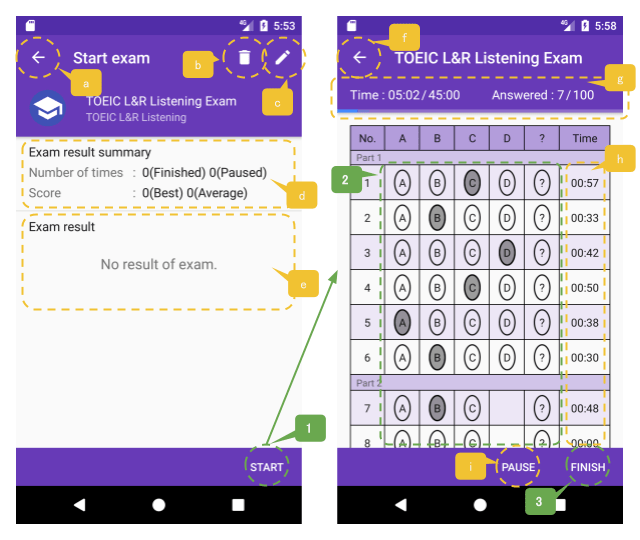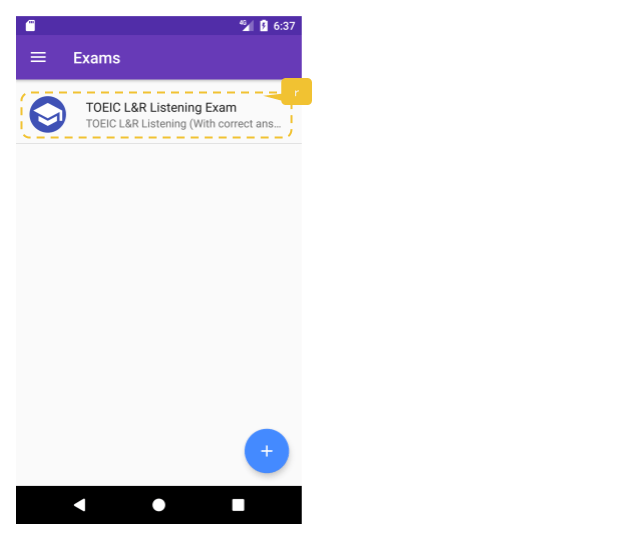(a) It returns to the first screen.
(b) Delete the exam. All results of this exam will also be deleted at this time.
(c) Edit the exam. If the result of this exam exists, you can not change the answer sheet.
(d) This is the area to show the exam result summary.
(e) This is the area to show a list of exam results.
(f) Pause the exam and return to [Start exam] screen. The exam is paused and shown in the list of exam results. You can resume the exam by tapping the paused exam.
(g) The progress of the exam is shown.
(h) The elapsed time for each question is shown.
(i) You can pause the exam by tapping [PAUSE] button. When paused, [PAUSE] button changes to [RESUME] button. Tap [RESUME] button to resume the exam.
(j) Close [Edit correct answer] screen.
(k) The name “answer sheet name (with correct answer)” is shown. You can change the name.
(l) The score is shown.
(m) You can edit the correct answer again by tapping the [SCORE] button.
(n) The exam result summary is shown. The number of exam runs, average score and best score are shown. The score are also shown in chart.
(o) The exam results are listed. If you tap it, you can check the results of the exam.
(p) Delete the exam result.
(q) By tapping the [START] button, you can try again.
(r) The created exams are listed.

By selecting (b)[Copy source answer sheet], you can edit based on the prepared answer sheet.

(2) Enter [Description] of the answer sheet.

(3) Select [Mark style].

You can select [Horizontal oval], [Vertical oval], [Horizontal bracket] or [Vertical bracket].

(4) Select [Letters]. That is letters to select the question.

You can select [Uppercase], [Lowercase] or [Numeric].

(5) Enter [Time limit(minute)].

(6) Enter the start number and end number of [Question number] that defines the question format. The start number usually starts with “1”.

You can add [Question Number] to be defined by tapping (c)[ADD QUESTION] button. You can delete the previous definition by tapping the (e)[REMOVE QUESTION] button. In this case the starting number is the previous end number plus one.

(7) Enter [Number of choices].

(8) Select [Selection type].

You can select [single] or [multiple].

(9) Enter [Group name].

[Group name] is optional. When it is set, it is shown on the answer sheet.

(10) Tap [SAVE] button to save the settings.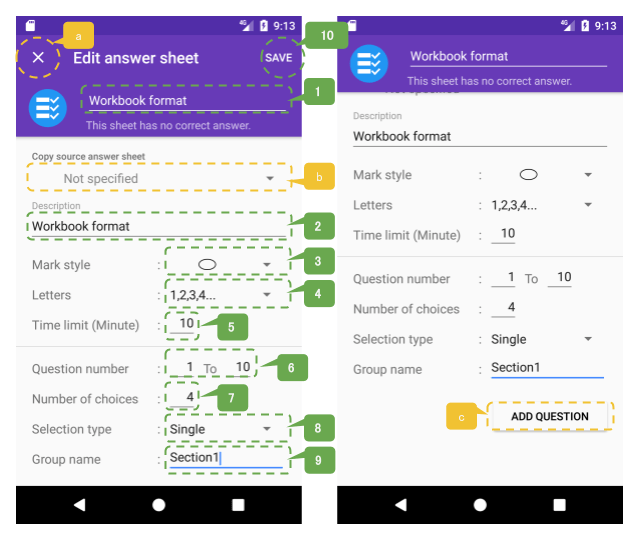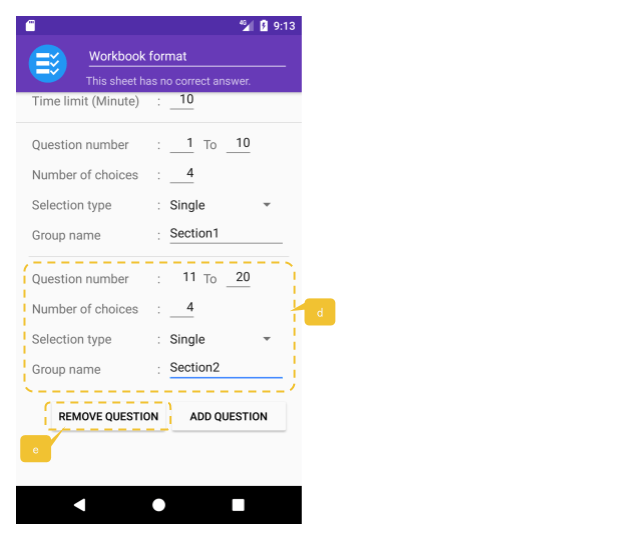(a) Close [Edit answer sheet] screen.
(b) When you select [Copy source answer sheet], the contents of the selected answer sheet will be copied.
(c) By tapping [ADD QUESTION] button, (d) the part defining questions is added.
(d) This area defines the added questions.
(e) When you tap [REMOVE QUESTION] button, the area that defines the previous questions is deleted.

### Other functions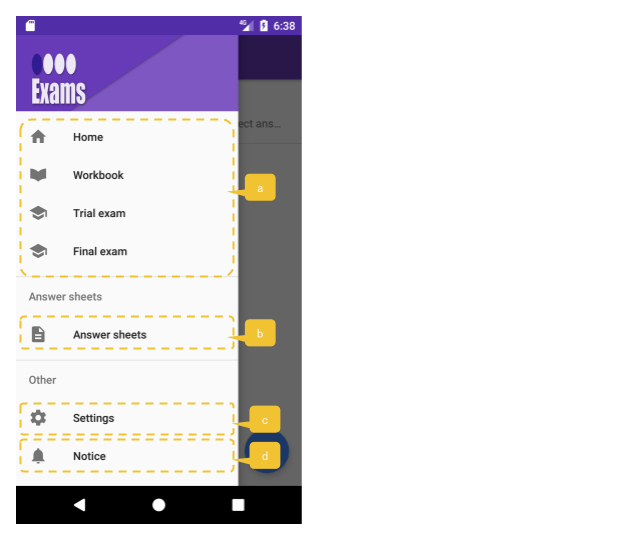(a) It is a filter of the exams list screen. Shows the exams of the selected category.
(b) A list of answer sheets is shown.
(c) [Settings] screen is shown.
(d) [Notice] screen is shown.

##### A list of answer sheets(a) A list of answer sheets is shown. Tap the item to display [Detail answer sheet] screen.
(b) Tap the [+] button to create a new answer sheet.
(c) Close [Detail answer sheet] screen.
(d) [Edit answer sheet] screen is shown.
(e) Select [Delete] to delete the answer sheet. Select [Create / Edit correct answer] to create / edit the correct answer. Select [Delete Correct Answer] to delete the correct answer.

##### Setting(a) Initialize all of the data. Delete all created data and data to the initial state.

##### Notice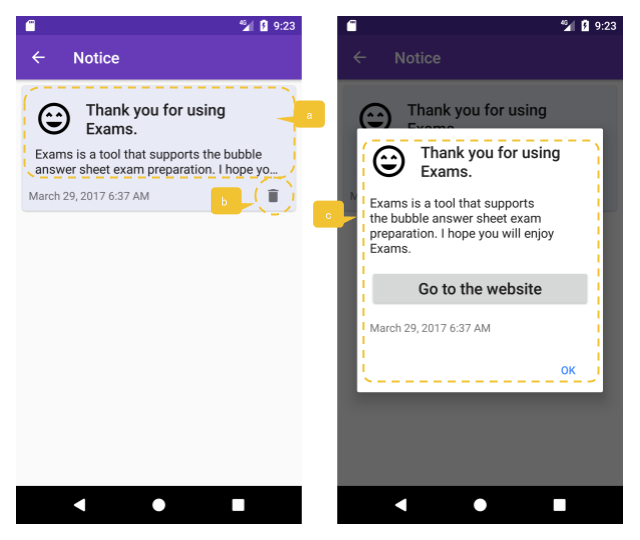(a) Notice list is shown. Tap to show (c) details.
(b) Delete the notice.
(c) Notice details are shown.